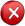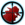Calculate the percentage of any number out of any number.Great little tool to have on hand.

External Mirror 1Report Broken LinkReport Spyware

# Function Grapher

Function Grapher is an easy-to-use software for 2D, 2.5D and 3D function graphing, animation and table data visualization.

# Percent

Calculate the percentage of any number out of any number. Add percentage to an amount or calculate a discount percentage. Free math homework help from basic math to algebra, geometry and beyond. Students, teachers, parents, and everyone can find solutions

# Math Calculator

To calculate expression, derivative, root, extremum, integral.To calculate expression, derivative, root, extremum, integral.To calculate expression, derivative, root, extremum, integral.